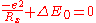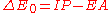xHarpoon reactionEncyclopedia
Harpoon reactions are a type of chemical reaction
Chemical reaction
A chemical reaction is a process that leads to the transformation of one set of chemical substances to another. Chemical reactions can be either spontaneous, requiring no input of energy, or non-spontaneous, typically following the input of some type of energy, such as heat, light or electricity...

between two substances one of them prone to form a cation, generally a metal
Metal
A metal , is an element, compound, or alloy that is a good conductor of both electricity and heat. Metals are usually malleable and shiny, that is they reflect most of incident light...

, and the other one prone to form an anion, generally a halogen
Halogen
The halogens or halogen elements are a series of nonmetal elements from Group 17 IUPAC Style of the periodic table, comprising fluorine , chlorine , bromine , iodine , and astatine...

.

Their main feature is that these reactions, unlike most reactions, have steric factor
Steric factor
Steric factor, P is a term used in collision theory.It is defined as the ratio between the experimental value of the rate constant and the one predicted by collision theory. It can also be defined as the ratio between the preexponential factor and the collision frequency, and it is most often...

s greater than unity, that is, they take place faster
Reaction rate
The reaction rate or speed of reaction for a reactant or product in a particular reaction is intuitively defined as how fast or slow a reaction takes place...

than predicted by collision theory
Collision theory
Collision theory is a theory proposed by Max Trautz and William Lewis in 1916 and 1918, that qualitatively explains how chemical reactions occur and why reaction rates differ for different reactions. For a reaction to occur the reactant particles must collide. Only a certain fraction of the total...

. This is explained by the fact that the colliding particles have greater cross sections
Cross section (physics)
A cross section is the effective area which governs the probability of some scattering or absorption event. Together with particle density and path length, it can be used to predict the total scattering probability via the Beer-Lambert law....

than the pure geometrical ones calculated from their radii, because when the particles are close enough, an electron "jumps" (therefore the name) from one of the particles to the other one, forming an anion and a cation which subsequently attract each other. Harpoon reactions usually take place in the gas
Gas
Gas is one of the three classical states of matter . Near absolute zero, a substance exists as a solid. As heat is added to this substance it melts into a liquid at its melting point , boils into a gas at its boiling point, and if heated high enough would enter a plasma state in which the electrons...

phase, but they are also possible in condensed media.

The calculated rate constant can be improved by using a better estimation of the steric factor. A rough approximation is that the largest separation Rx at which charge transfer can take place on energetic grounds, can be estimated from the solution of the following equation that determines the largest distance at which the Coulombic attraction between the two oppositely charged ions is sufficient to provide the energy ΔE0With, where IP is the ionization potential
Ionization potential
The ionization energy of a chemical species, i.e. an atom or molecule, is the energy required to remove an electron from the species to a practically infinite distance. Large atoms or molecules have a low ionization energy, while small molecules tend to have higher ionization energies.The property...

of the metal and EA is the electron affinity
Electron affinity
The Electron affinity of an atom or molecule is defined as the amount of energy released when an electron is added to a neutral atom or molecule to form a negative ion....

of the halogen.

## Examples of harpoon reactions

• Generically: Rg + X2 + hν → RgX + X, where Rg is a rare gas and X is a halogen
• Ba...FCH3 + hν → BaF(*) + CH3
• K + CH3I → KI + CH3